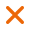# Pulley Formula

Formula D1 x N1 = D2 ÷ N2

D1 = Tail-Pulley diameter
N1 = revolutions of driving pulley
D2 = Drive-Pulley diameter
N2 = revolutions of driven pulley

*Knowing the speed of the fan and tail-pulleys, want to know the size of the drive-pulley

Set :
 - Fan Speed = 900 RPM - Tail-Pulley 8" - Drive-Pulley? - Motor 4P (1,400 RPM)

method :
 = D1 x N1 = D2 N2 = 8" x 900 = D2 1,400 = 7,200 = D2 1,400 = 5.14 นิ้ว